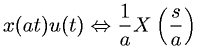Equations > Signal Processing > Laplace Transform Properties > Laplace transform time domain scaling property

### Laplace transform time domain scaling propertyLatex Code:

MathML Code:

 $x\left(\mathrm{at}\right)u\left(t\right)⇔\frac1aX\left(\fracsa\right)$

MathType 5.0: